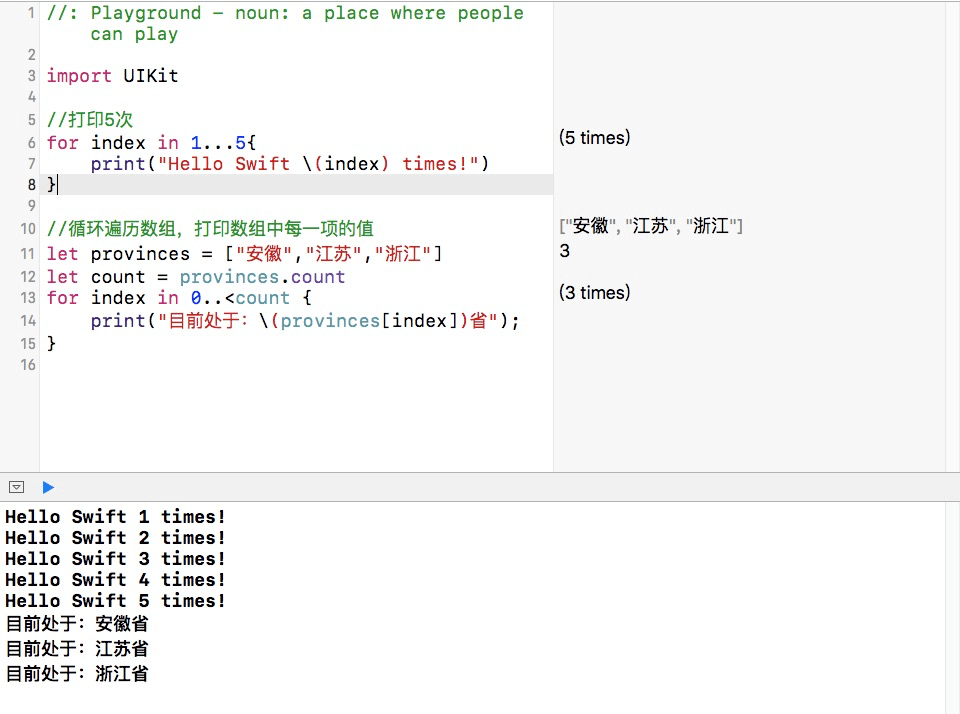# 常用的运算符

Swift支持大部分标准C语言的运算符，同时改进了许多特性来减少常规编码错误。比如，赋值运算符"="不返回值，来防止在想要判断相等运算符"=="的地方写成了赋值运算符"="导致的隐藏性错误。取消了自增"++"和自减"--"运算符，推荐使用复合赋值"+="和"-="。下面是一些常用运算符的使用示例：

``````let one = 5
var two = 10
two = one //赋值运算符
two = one + two //算术运算符
two += one //复合赋值运算符
let mod = two % one //求余运算符
one == two //关系运算符
let who = (two > one) ? true : false //三元运算符
let me = (two / one == 2) ? true : false
who && !me //逻辑运算符
``````# 区间运算符

• 闭合区间运算符(from...to)定义了一个从from到to(包括from和two)的所有整数值的区间。闭合区间运算符在for循环一个连续区间值的时候非常有用。
• 半闭区间(from..<to)定义了一个从from到to但不包含to的区间。之所以称之为半闭区间，就是因为它包含第一个值而不包含最后的值。半闭区间的实用性在于，当你使用一个以0开始的数组时，非常方便地从0数到数组的长度。具体的演示代码如下：
``````//打印5次
for index in 1...5{
print("Hello Swift \(index) times!")
}

//循环遍历数组，打印数组中每一项的值
let provinces = ["安徽","江苏","浙江"]
let count = provinces.count
for index in 0..<count {
print("目前处于：\(provinces[index])省");
}
``````